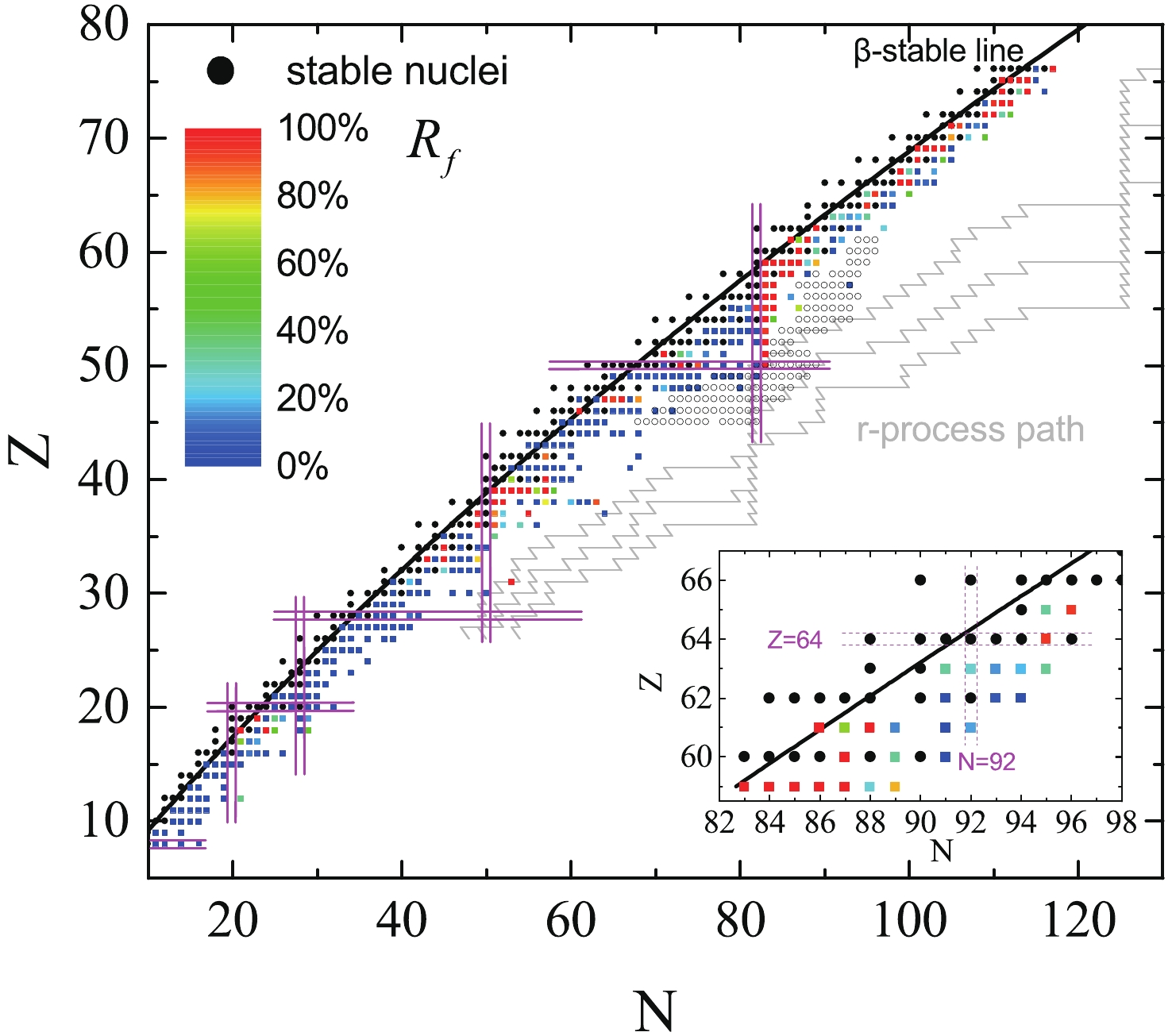# The contribution of the first forbidden transitions to the nuclear β−-decay half-life

• $\beta$-decay half-life is a key quantity for nuclear structure and nucleosynthesis studies. There exist large uncertainties in the contributions of allowed and forbidden transitions to the total $\beta$-decay life, which limits the resolution of the predicted $\beta$-decay half-life. We systematically study the contribution of the first forbidden (FF) transitions to the $\beta^{-}$-decay half-life, and quantify it with a formula based on simple physics considerations. We also propose a new formula for calculation of the $\beta^{-}$-decay half-life that includes the FF contribution. It is shown that the inclusion of the contribution of FF transitions significantly improves the precision of calculations of the $\beta^{-}$-decay half-life. By fitting of the RQRPA results for neutron-rich $Z = 47$, 57 isotopes and $N = 80$, 94 isotones, the formula for the contribution of the FF transitions gives similar results as the RQRPA calculations. However, because of limited experimental data for the branching ratios of unstable nuclei, the fit parameters are not fully constrained. Therefore, the proposed formula for the $\beta^{-}$-decay half-life is more suitable for calculations of half-lives than of the FF contributions. The formula could be used to predict the $\beta^{-}$-decay half-life in nuclear structure studies as well as nucleosynthesis calculations in stars.
••Get Citation
Ji-Lin You, Xiao-Ping Zhang, Qi-Jun Zhi, Zhong-Zhou Ren and Qing-Dong Wu. The contribution of the first forbidden transitions to the nuclear β-decay half-life[J]. Chinese Physics C, 2019, 43(11): 114104. doi: 10.1088/1674-1137/43/11/114104
Ji-Lin You, Xiao-Ping Zhang, Qi-Jun Zhi, Zhong-Zhou Ren and Qing-Dong Wu. The contribution of the first forbidden transitions to the nuclear β-decay half-life[J]. Chinese Physics C, 2019, 43(11): 114104.Milestone
Revised: 2019-08-16
Article Metric

Article Views(1082)
Cited by(0)
Policy on re-use
To reuse of subscription content published by CPC, the users need to request permission from CPC, unless the content was published under an Open Access license which automatically permits that type of reuse.
###### 通讯作者: 陈斌, bchen63@163.com
• 1.

沈阳化工大学材料科学与工程学院 沈阳 110142

Title:
Email:

## The contribution of the first forbidden transitions to the nuclear β−-decay half-life

• 1. School of Physics and Electronic Science, Guizhou Normal University, Guiyang 550001, China
• 2. State Key Laboratory of Lunar and Planetary Sciences, Macau University of Science and Technology, Macau 999078, China
• 3. Guizhou Provincial Key Laboratory of Radio Astronomy and Data Processing, Guizhou Normal University, Guiyang 550001, China
• 4. School of Physics Science and Engineering, Tongji University, Shanghai 200092, China

Abstract: $\beta$-decay half-life is a key quantity for nuclear structure and nucleosynthesis studies. There exist large uncertainties in the contributions of allowed and forbidden transitions to the total $\beta$-decay life, which limits the resolution of the predicted $\beta$-decay half-life. We systematically study the contribution of the first forbidden (FF) transitions to the $\beta^{-}$-decay half-life, and quantify it with a formula based on simple physics considerations. We also propose a new formula for calculation of the $\beta^{-}$-decay half-life that includes the FF contribution. It is shown that the inclusion of the contribution of FF transitions significantly improves the precision of calculations of the $\beta^{-}$-decay half-life. By fitting of the RQRPA results for neutron-rich $Z = 47$, 57 isotopes and $N = 80$, 94 isotones, the formula for the contribution of the FF transitions gives similar results as the RQRPA calculations. However, because of limited experimental data for the branching ratios of unstable nuclei, the fit parameters are not fully constrained. Therefore, the proposed formula for the $\beta^{-}$-decay half-life is more suitable for calculations of half-lives than of the FF contributions. The formula could be used to predict the $\beta^{-}$-decay half-life in nuclear structure studies as well as nucleosynthesis calculations in stars.

### HTML2.   The formula for the FF contribution in $\,{{\beta^{-}}}$-decay3.   ${{\beta^{-}}}$-decay half-life including the FF transitions
Reference (43)
PDF查看关注分享

Top

### 目录/DownLoad:  Full-Size Img  PowerPoint